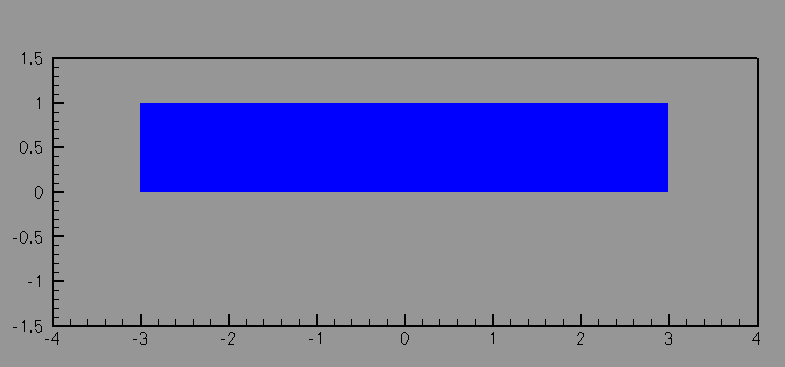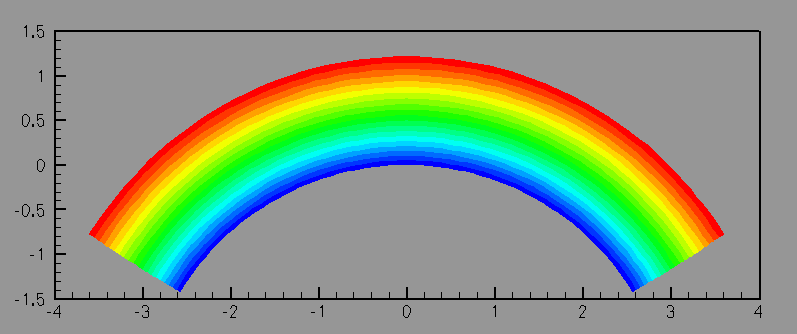Thermoelasticity: Combining the Heat equation and non-linear Solid MechanicsUndeformed configuration of an elastic block.

We consider the uniform steady thermal expansion of an elastic body that is differentially heated. The top surface is heated and the bottom surface is maintained at the reference temperature, which leads to a uniform temperature gradient throughout the material. The material expands more near the upper surface than near the lower surface, deforming the initially rectangular block into an curved configuration.Deformed elastica when the top surface is heated and the lower surface is maintained at the reference temperature. The contours indicate the temperature of the body.

# The driver code

The driver code for this example is given below:

//LIC// ====================================================================
//LIC// This file forms part of oomph-lib, the object-oriented,
//LIC// multi-physics finite-element library, available
//LIC// at http://www.oomph-lib.org.
//LIC//
//LIC// Copyright (C) 2006-2022 Matthias Heil and Andrew Hazel
//LIC//
//LIC// This library is free software; you can redistribute it and/or
//LIC// modify it under the terms of the GNU Lesser General Public
//LIC// version 2.1 of the License, or (at your option) any later version.
//LIC//
//LIC// This library is distributed in the hope that it will be useful,
//LIC// but WITHOUT ANY WARRANTY; without even the implied warranty of
//LIC// MERCHANTABILITY or FITNESS FOR A PARTICULAR PURPOSE. See the GNU
//LIC// Lesser General Public License for more details.
//LIC//
//LIC// You should have received a copy of the GNU Lesser General Public
//LIC// License along with this library; if not, write to the Free Software
//LIC// Foundation, Inc., 51 Franklin Street, Fifth Floor, Boston, MA
//LIC// 02110-1301 USA.
//LIC//
//LIC// The authors may be contacted at oomph-lib@maths.man.ac.uk.
//LIC//
//LIC//====================================================================
//Driver for a multi-physics problem that couples the
//unsteady heat equation to the equations of large-displacement solid
//mechanics
//and and elements.
#include "generic.h"
#include "solid.h"
// The mesh is our standard rectangular quadmesh
// Use the oomph and std namespaces
using namespace oomph;
using namespace std;
//=====================================================================
/// A class that solves the equations of steady thermoelasticity by
/// combining the UnsteadyHeat and PVD equations into a single element.
/// A temperature-dependent growth term is added to the PVD equations by
//======================class definition==============================
template<unsigned DIM>
class QThermalPVDElement : public virtual QPVDElement<DIM,3>,
{
private:
/// Pointer to a private data member, the thermal expansion coefficient
double* Alpha_pt;
/// The static default value of Alpha
public:
/// Constructor: call the underlying constructors and
/// initialise the pointer to Alpha to point
/// to the default value of 1.0.
QThermalPVDElement() : QPVDElement<DIM,3>(),
{
}
/// The required number of values stored at the nodes is the sum of the
/// required values of the two single-physics elements. Note that this step is
/// generic for any multi-physics element of this type.
unsigned required_nvalue(const unsigned &n) const
QPVDElement<DIM,3>::required_nvalue(n));}
/// Access function for the thermal expansion coefficient (const version)
const double &alpha() const {return *Alpha_pt;}
/// Access function for the pointer to the thermal expansion coefficientr
double* &alpha_pt() {return Alpha_pt;}
/// Overload the standard output function with the broken default
void output(ostream &outfile) {FiniteElement::output(outfile);}
/// Output function:
/// Output x, y, u, v, p, theta at Nplot^DIM plot points
// Start of output function
void output(ostream &outfile, const unsigned &nplot)
{
//vector of local coordinates
Vector<double> s(DIM);
Vector<double> xi(DIM);
outfile << this->tecplot_zone_string(nplot);
// Loop over plot points
unsigned num_plot_points=this->nplot_points(nplot);
for (unsigned iplot=0;iplot<num_plot_points;iplot++)
{
// Get local coordinates of plot point
this->get_s_plot(iplot,nplot,s);
// Get the Lagrangian coordinate
this->interpolated_xi(s,xi);
// Output the position of the plot point
for(unsigned i=0;i<DIM;i++)
{outfile << this->interpolated_x(s,i) << " ";}
// Output the temperature (the advected variable) at the plot point
outfile << this->interpolated_u_ust_heat(s) << std::endl;
}
outfile << std::endl;
// Write tecplot footer (e.g. FE connectivity lists)
this->write_tecplot_zone_footer(outfile,nplot);
} //End of output function
/// C-style output function: Broken default
void output(FILE* file_pt)
{FiniteElement::output(file_pt);}
/// C-style output function: Broken default
void output(FILE* file_pt, const unsigned &n_plot)
{FiniteElement::output(file_pt,n_plot);}
/// Output function for an exact solution: Broken default
void output_fct(ostream &outfile, const unsigned &Nplot,
exact_soln_pt)
{FiniteElement::output_fct(outfile,Nplot,exact_soln_pt);}
/// Output function for a time-dependent exact solution:
/// Broken default.
void output_fct(ostream &outfile, const unsigned &Nplot,
const double& time,
exact_soln_pt)
{
FiniteElement::
output_fct(outfile,Nplot,time,exact_soln_pt);
}
/// Compute norm of solution: use the version in the unsteady heat
/// class
void compute_norm(double& el_norm)
{
}
/// Validate against exact solution at given time
/// Solution is provided via function pointer.
/// Plot at a given number of plot points and compute L2 error
/// and L2 norm of velocity solution over element
/// Call the broken default
void compute_error(ostream &outfile,
const double& time,
double& error, double& norm)
{FiniteElement::compute_error(outfile,exact_soln_pt,
time,error,norm);}
/// Validate against exact solution.
/// Solution is provided via function pointer.
/// Plot at a given number of plot points and compute L2 error
/// and L2 norm of velocity solution over element
/// Call the broken default
void compute_error(ostream &outfile,
double& error, double& norm)
{FiniteElement::compute_error(outfile,exact_soln_pt,error,norm);}
/// to be temperature-dependent.
void get_isotropic_growth(const unsigned& ipt, const Vector<double> &s,
const Vector<double>& xi, double &gamma) const
{
//The growth is the undeformed coefficient plus linear thermal
//expansion
gamma = 1.0 + (*Alpha_pt)*this->interpolated_u_ust_heat(s);
}
/// Calculate the contribution to the residual vector.
/// We assume that the vector has been initialised to zero
/// before this function is called.
void fill_in_contribution_to_residuals(Vector<double> &residuals)
{
//Call the residuals of the advection-diffusion eqautions
fill_in_contribution_to_residuals(residuals);
//Call the residuals of the Navier-Stokes equations
PVDEquations<DIM>::
fill_in_contribution_to_residuals(residuals);
}
/// Compute the element's residual Vector and the jacobian matrix
/// We assume that the residuals vector and jacobian matrix have been
/// initialised to zero before calling this function
void fill_in_contribution_to_jacobian(Vector<double> &residuals,
DenseMatrix<double> &jacobian)
{
//Just call standard finite difference for a SolidFiniteElement so
//that variations in the nodal positions are taken into account
SolidFiniteElement::fill_in_contribution_to_jacobian(residuals,jacobian);
}
};
//=========================================================================
/// Set the default physical value to be one
//=========================================================================
template<>
//======start_of_namespace============================================
/// Namespace for the physical parameters in the problem
//====================================================================
{
/// Thermal expansion coefficient
double Alpha=0.0;
/// Young's modulus for solid mechanics
double E = 1.0; // ADJUST
/// Poisson ratio for solid mechanics
double Nu = 0.3; // ADJUST
/// We need a constitutive law for the solid mechanics
ConstitutiveLaw* Constitutive_law_pt;
} // end_of_namespace
/// ///////////////////////////////////////////////////////////////////
/// ///////////////////////////////////////////////////////////////////
/// ///////////////////////////////////////////////////////////////////
//====== start_of_problem_class=======================================
/// 2D Thermoelasticity problem on rectangular domain, discretised
/// with refineable elements. The specific type
/// of element is specified via the template parameter.
//====================================================================
template<class ELEMENT>
class ThermalProblem : public Problem
{
public:
/// Constructor
/// Destructor. Empty
/// Update the problem specs before solve (empty)
/// Update the problem after solve (empty)
/// Doc the solution.
void doc_solution();
/// Overloaded version of the problem's access function to
/// the mesh. Recasts the pointer to the base Mesh object to
/// the actual mesh type.
{
Problem::mesh_pt());
}
private:
/// DocInfo object
DocInfo Doc_info;
}; // end of problem class
//========================================================================
/// Constructor for Convection problem
//========================================================================
template<class ELEMENT>
{
// Set output directory
Doc_info.set_directory("RESLT");
// # of elements in x-direction
unsigned n_x=8;
// # of elements in y-direction
unsigned n_y=8;
// Domain length in x-direction
double l_x=3.0;
// Domain length in y-direction
double l_y=1.0;
// Build a standard rectangular quadmesh
Problem::mesh_pt() =
// Set the boundary conditions for this problem: All nodes are
// free by default -- only need to pin the ones that have Dirichlet
// conditions here
{
//The temperature is prescribed on the lower boundary
unsigned n_boundary_node = mesh_pt()->nboundary_node(0);
for(unsigned n=0;n<n_boundary_node;n++)
{
//Get the pointer to the node
Node* nod_pt = mesh_pt()->boundary_node_pt(0,n);
//Pin the temperature at the node
nod_pt->pin(0);
//Set the temperature to 0.0 (cooled)
nod_pt->set_value(0,0.0);
}
//The temperature is prescribed on the upper boundary
n_boundary_node = mesh_pt()->nboundary_node(2);
for(unsigned n=0;n<n_boundary_node;n++)
{
Node* nod_pt = mesh_pt()->boundary_node_pt(2,n);
//Pin the temperature at the node
nod_pt->pin(0);
//Set the temperature to 1.0 (heated)
nod_pt->set_value(0,1.0);
}
//The horizontal-position is fixed on the vertical boundary (symmetry)
n_boundary_node = mesh_pt()->nboundary_node(1);
for(unsigned n=0;n<n_boundary_node;n++)
{
static_cast<SolidNode*>(mesh_pt()->boundary_node_pt(1,n))->pin_position(0);
}
//We need to completely fix the lower-right corner of the block to
//prevent vertical rigid-body motions
static_cast<SolidNode*>(mesh_pt()->boundary_node_pt(1,0))->pin_position(1);
}
// Complete the build of all elements so they are fully functional
// Loop over the elements to set up element-specific
// things that cannot be handled by the (argument-free!) ELEMENT
// constructor.
unsigned n_element = mesh_pt()->nelement();
for(unsigned int i=0;i<n_element;i++)
{
// Upcast from GeneralsedElement to the present element
ELEMENT *el_pt = dynamic_cast<ELEMENT*>(mesh_pt()->element_pt(i));
// Set the coefficient of thermal expansion
el_pt->alpha_pt() = &Global_Physical_Variables::Alpha;
// Set a constitutive law
el_pt->constitutive_law_pt() =
}
// Setup equation numbering scheme
cout <<"Number of equations: " << assign_eqn_numbers() << endl;
} // end of constructor
//========================================================================
/// Doc the solution
//========================================================================
template<class ELEMENT>
{
//Declare an output stream and filename
ofstream some_file;
char filename;
// Number of plot points: npts x npts
unsigned npts=5;
// Output solution
//-----------------
sprintf(filename,"%s/soln%i.dat",Doc_info.directory().c_str(),
Doc_info.number());
some_file.open(filename);
mesh_pt()->output(some_file,npts);
some_file.close();
Doc_info.number()++;
} // end of doc
//=====================================================================
/// Driver code for 2D Thermoelasticity problem
//====================================================================
int main(int argc, char **argv)
{
// "Big G" Linear constitutive equations:
new GeneralisedHookean(&Global_Physical_Variables::Nu,
//Construct our problem
unsigned n_steps = 11;
//If we have additional command line arguemnts, take fewer steps
if(argc > 1) {n_steps = 2;}
for(unsigned i=0;i<n_steps;i++)
{
//Increase the thermal expansion coefficient
//Perform a single steady newton solve
problem.newton_solve();
//Document the solution
problem.doc_solution();
}
} // end of main
A class that solves the equations of steady thermoelasticity by combining the UnsteadyHeat and PVD eq...
Definition: thermo.cc:54
const double & alpha() const
Access function for the thermal expansion coefficient (const version)
Definition: thermo.cc:81
QThermalPVDElement()
Constructor: call the underlying constructors and initialise the pointer to Alpha to point to the def...
Definition: thermo.cc:67
void get_isotropic_growth(const unsigned &ipt, const Vector< double > &s, const Vector< double > &xi, double &gamma) const
Definition: thermo.cc:181
unsigned required_nvalue(const unsigned &n) const
The required number of values stored at the nodes is the sum of the required values of the two single...
Definition: thermo.cc:76
void compute_error(ostream &outfile, FiniteElement::UnsteadyExactSolutionFctPt exact_soln_pt, const double &time, double &error, double &norm)
Validate against exact solution at given time Solution is provided via function pointer....
Definition: thermo.cc:162
void fill_in_contribution_to_jacobian(Vector< double > &residuals, DenseMatrix< double > &jacobian)
Compute the element's residual Vector and the jacobian matrix We assume that the residuals vector and...
Definition: thermo.cc:205
void compute_norm(double &el_norm)
Compute norm of solution: use the version in the unsteady heat class.
Definition: thermo.cc:152
double * Alpha_pt
Pointer to a private data member, the thermal expansion coefficient.
Definition: thermo.cc:58
void output(ostream &outfile)
Overload the standard output function with the broken default.
Definition: thermo.cc:87
void fill_in_contribution_to_residuals(Vector< double > &residuals)
Calculate the contribution to the residual vector. We assume that the vector has been initialised to ...
Definition: thermo.cc:192
void output_fct(ostream &outfile, const unsigned &Nplot, FiniteElement::SteadyExactSolutionFctPt exact_soln_pt)
Output function for an exact solution: Broken default.
Definition: thermo.cc:133
static double Default_Physical_Constant_Value
The static default value of Alpha.
Definition: thermo.cc:61
double *& alpha_pt()
Access function for the pointer to the thermal expansion coefficientr.
Definition: thermo.cc:84
/////////////////////////////////////////////////////////////////// /////////////////////////////////...
Definition: thermo.cc:251
ThermalProblem()
Constructor.
Definition: thermo.cc:293
Definition: thermo.cc:268
Overloaded version of the problem's access function to the mesh. Recasts the pointer to the base Mesh...
Definition: thermo.cc:276
void doc_solution()
Doc the solution.
Definition: thermo.cc:382
void actions_before_newton_solve()
Update the problem specs before solve (empty)
Definition: thermo.cc:262
void actions_after_newton_solve()
Update the problem after solve (empty)
Definition: thermo.cc:265
DocInfo Doc_info
DocInfo object.
Definition: thermo.cc:285
~ThermalProblem()
Destructor. Empty.
Definition: thermo.cc:259
Namespace for the physical parameters in the problem.
Definition: thermo.cc:225
double E
Young's modulus for solid mechanics.
Definition: thermo.cc:230
ConstitutiveLaw * Constitutive_law_pt
We need a constitutive law for the solid mechanics.
Definition: thermo.cc:236
double Nu
Poisson ratio for solid mechanics.
Definition: thermo.cc:233
double Alpha
Thermal expansion coefficient.
Definition: thermo.cc:227
int main(int argc, char **argv)
Driver code for 2D Thermoelasticity problem.
Definition: thermo.cc:406